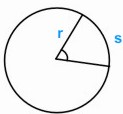HOME MATH DICTIONARY DOWNLOAD FEEDBACK DISCLAIMER
 Question: What is Radian Measure ? Answer: A radian is a unit of measure for angles. The angle formed at the center of a circle of radius r by an arc of length s is defined as s/r radians. It follows that 1 radian is formed by an arc of length r of a circle with radius r, and that a complete revolution is equal toMost calculators are programmed so that either radians (rad) or degrees can be selected.